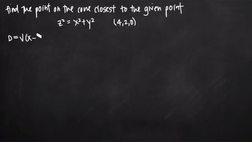PLAY PREVIEW

### Segments in this Video

#### Finding the Point on a Cone(00:56)

FREE PREVIEW

We are looking to find the point on the cone which is closest to a given point. It needs to be recognized as multivariable that is applied to optimize the problem. We must find the smallest possible distance between the coordinate point and the surface of the cone.

#### Optimizing the Cone Distance Formula(01:56)

The distance formula is in three variables and will give you the distance between two coordinate points in three-dimensional space. To optimize the equation, you must eliminate one of the variables and leaving only two variables. This will give a function that can be easily optimized.

#### Simplifying the Equation(01:42)

Square both sides to simplify the equation. Simplifying gives you the equation you are optimizing.

#### Taking Partial Derivatives of the Equation(00:48)

In order to optimize an equation, you take the partial derivatives of each variable in the equation. The goal to finding partial derivatives is find a critical point.

#### Finding the Coordinate Point on the Surface of the Cone(01:04)

To find the coordinate point that lies on the surface of the cones, plug in the critical point into the equation. There are two points on the surface of the cone that are equidistant from the given coordinate point.

#### The Second Derivative Test(02:15)

The second derivative test can be used to determine whether a given coordinate point is a minimum or a maximum on a surface.

#### Credits(00:00)

Credits

For additional digital leasing and purchase options contact a media consultant at 800-257-5126
(press option 3) or sales@films.com.

# Point on a cone closest to another point

Part of the Series : Integral Calc: Calculus 3
 3-Year Streaming Price: \$49.95

Share

### Description

This video tutorial works through math problems/equations that address topics in Calculus 3, Partial Derivatives. This specific tutorial addresses Point on a cone closest to another point.

Length: 9 minutes

Item#: BVL275744Closed Captioned